# MSBSHSE Solutions For Class 8 Maths Part 1 Chapter 4- Altitudes and Medians of a Triangle

MSBSHSE Solutions For Class 8 Maths Part 1 Chapter 4- Altitudes and Medians of a Triangle are available here. Our expert tutors at BYJU’S have developed the solutions to help students with their exam preparation to acquire good marks in Maths. If you wish to secure an excellent score then solving MSBSHSE Solutions for Class 8 is an utmost necessity. This chapter mainly discusses problems based on altitudes and medians. In order to help you understand and solve the problems easily, students can refer to Maharashtra State Board Class 8 Textbooks Part 1 Maths Solutions. The PDF of Maharashtra Board Solutions for Class 8 Maths Chapter 4 Altitudes and Medians of a Triangle is provided here. Students can easily download the pdf of Class 8 Chapter 4 in their respective links.

## Download the PDF of Maharashtra Board Solutions For Class 8 Maths Part 1 Chapter 4- Altitudes and Medians of a Triangle.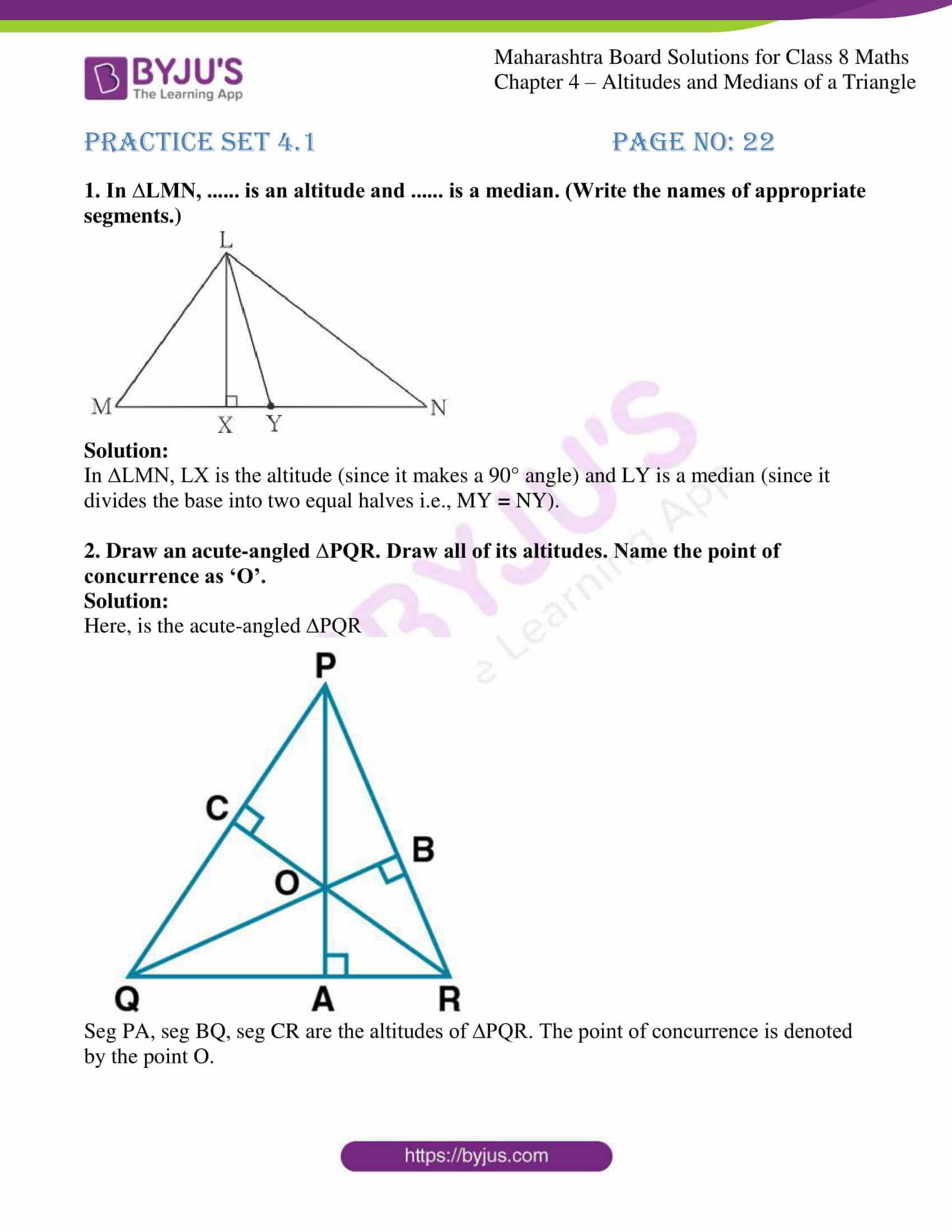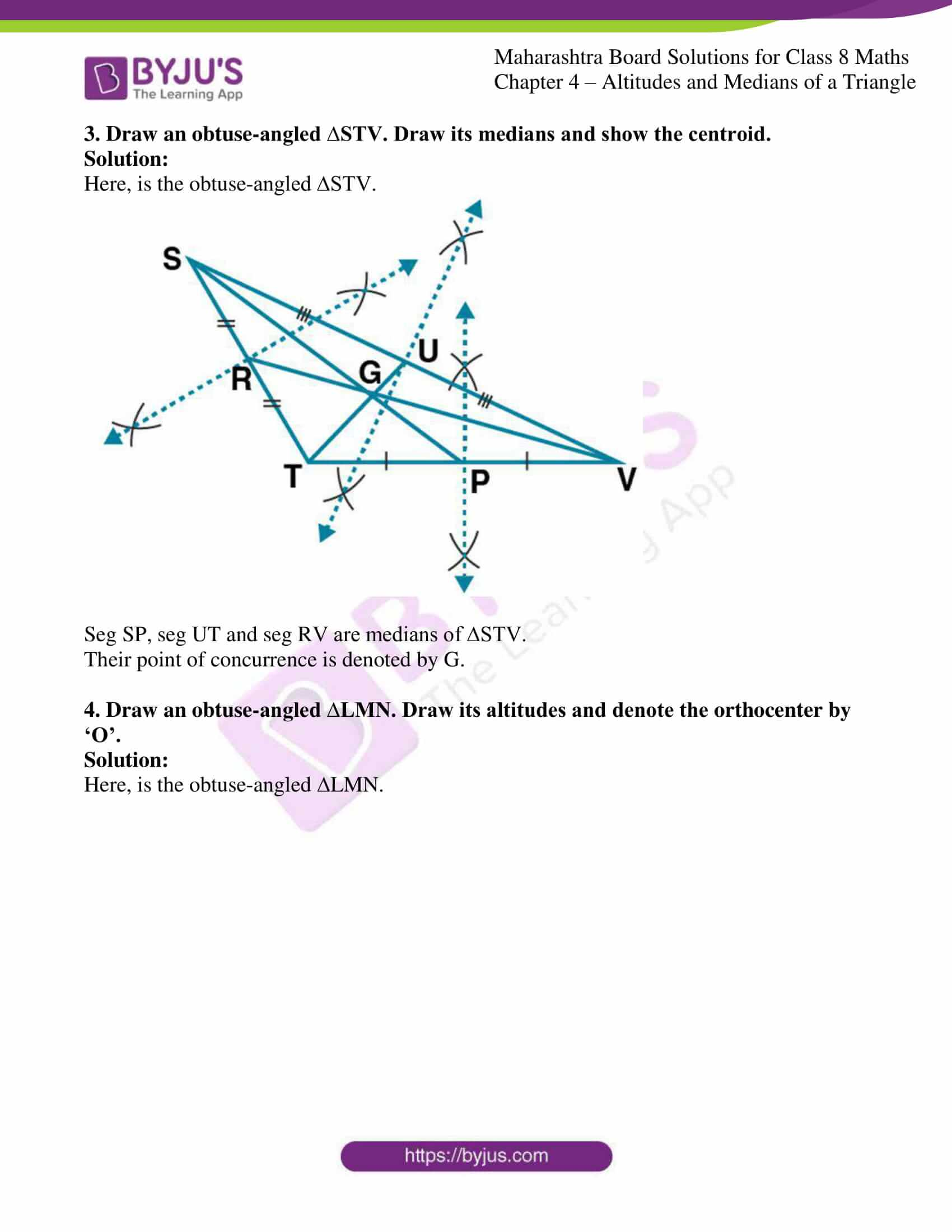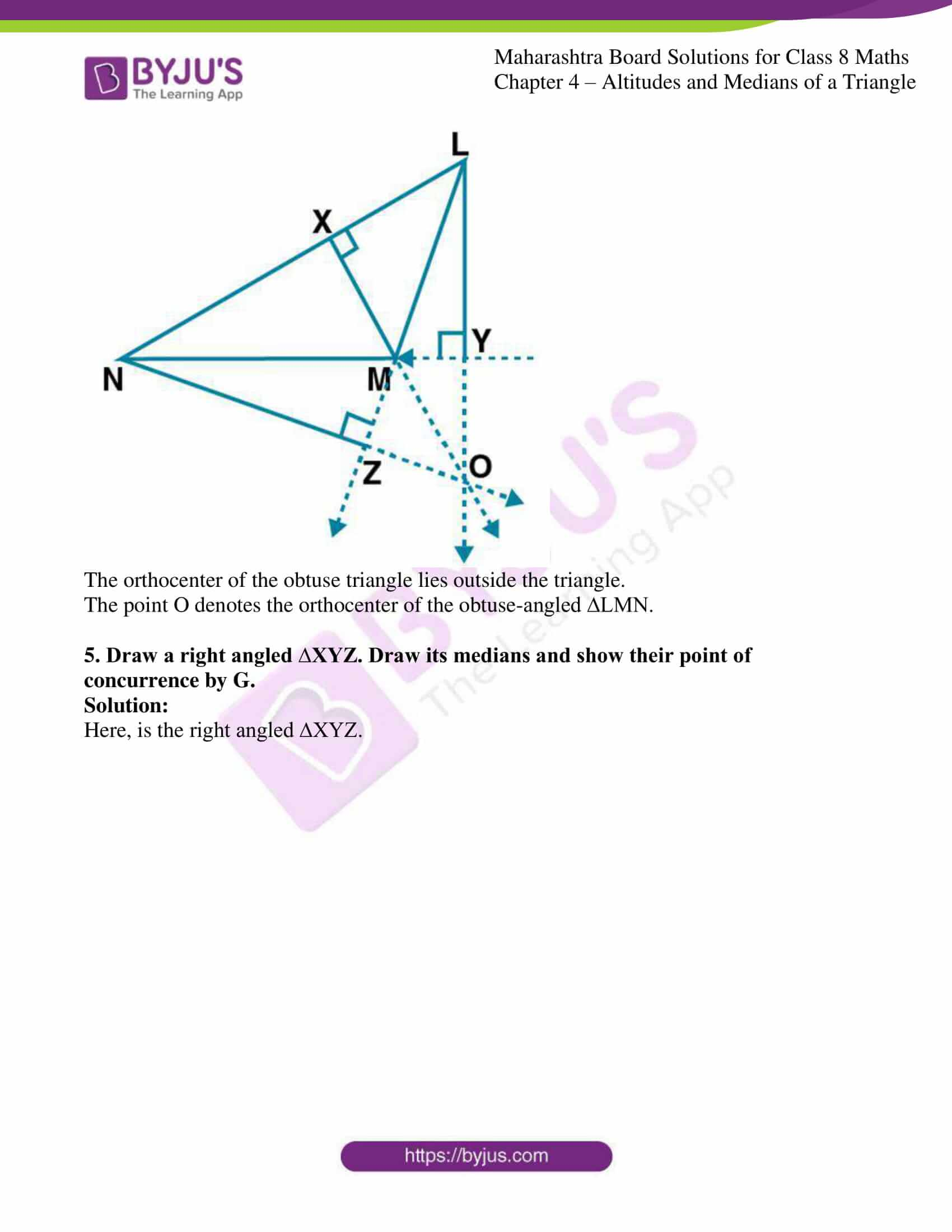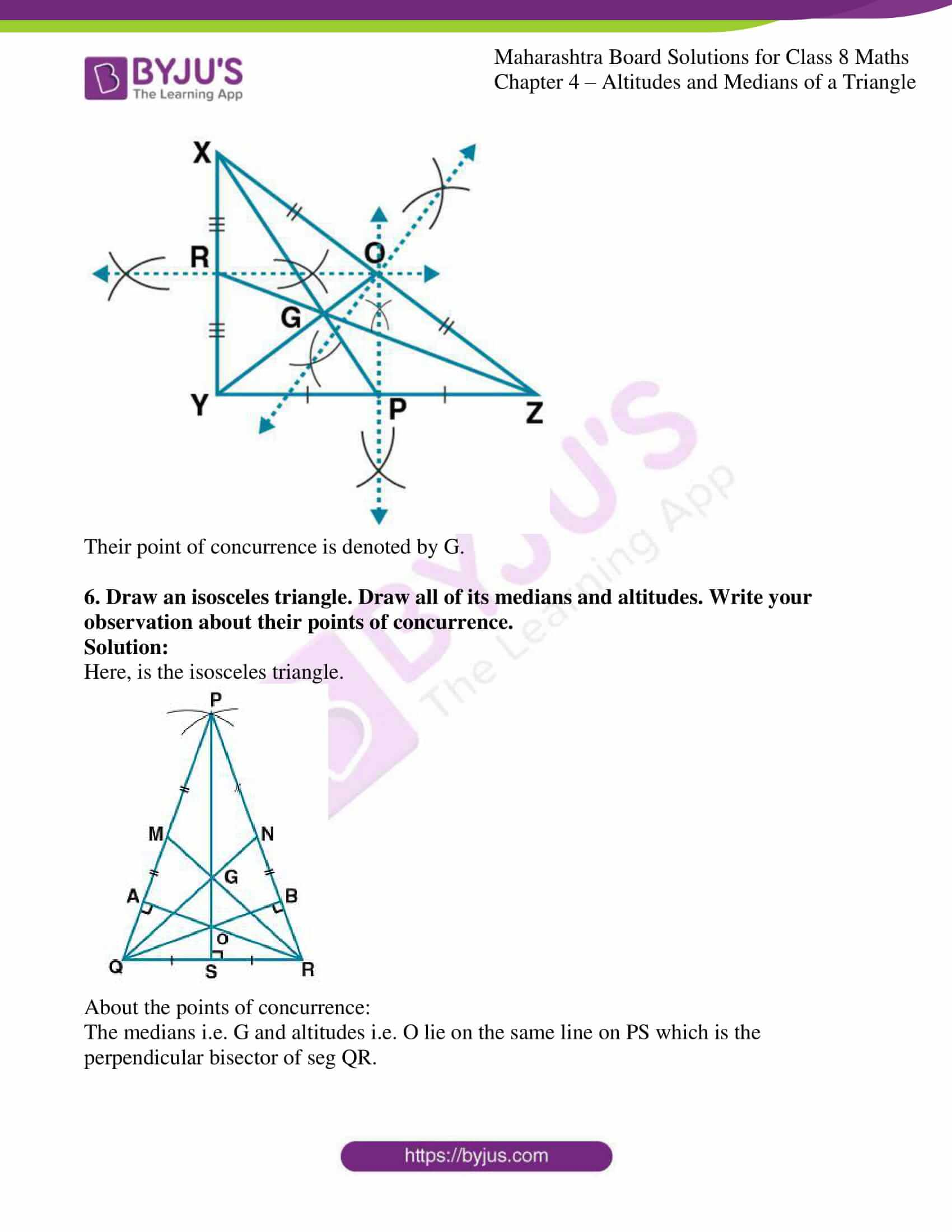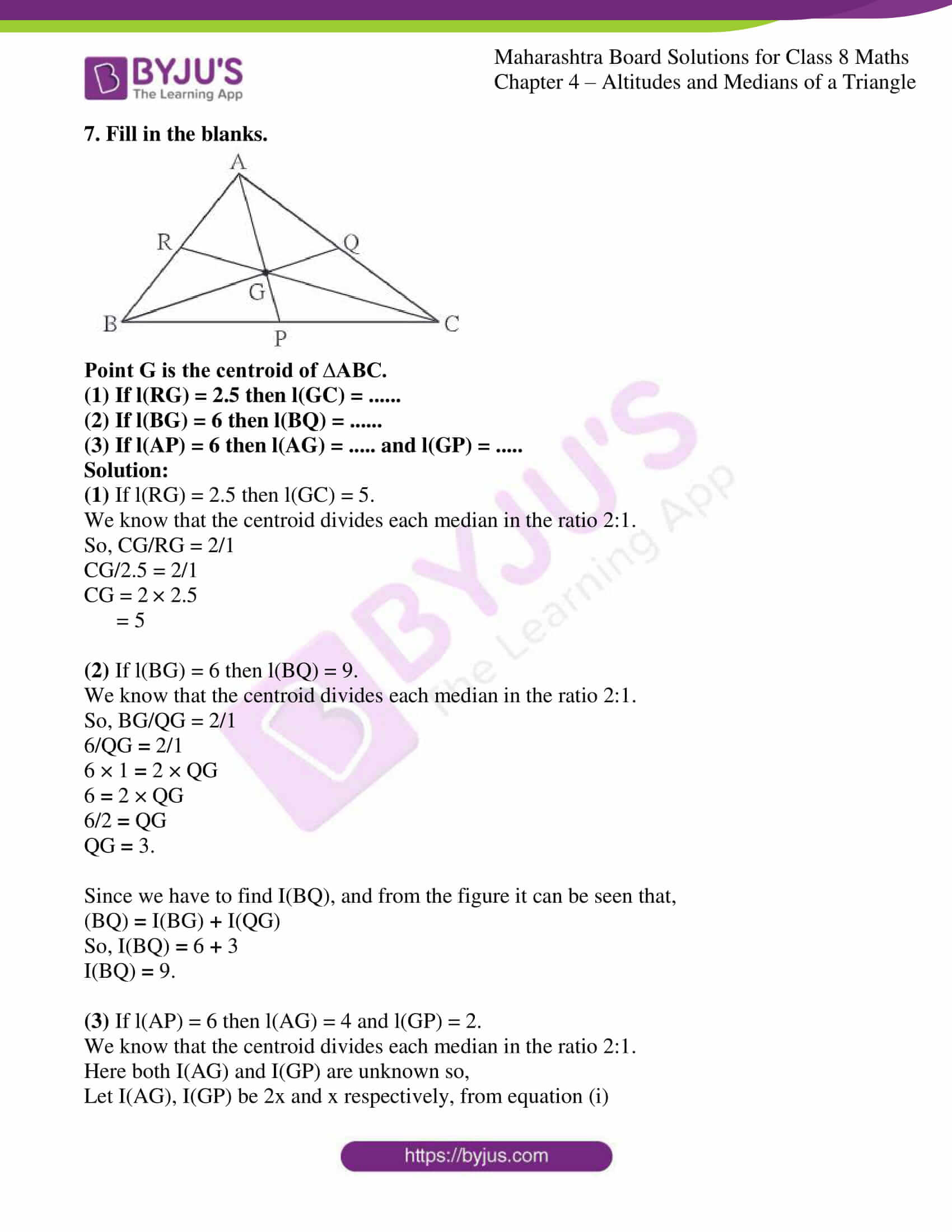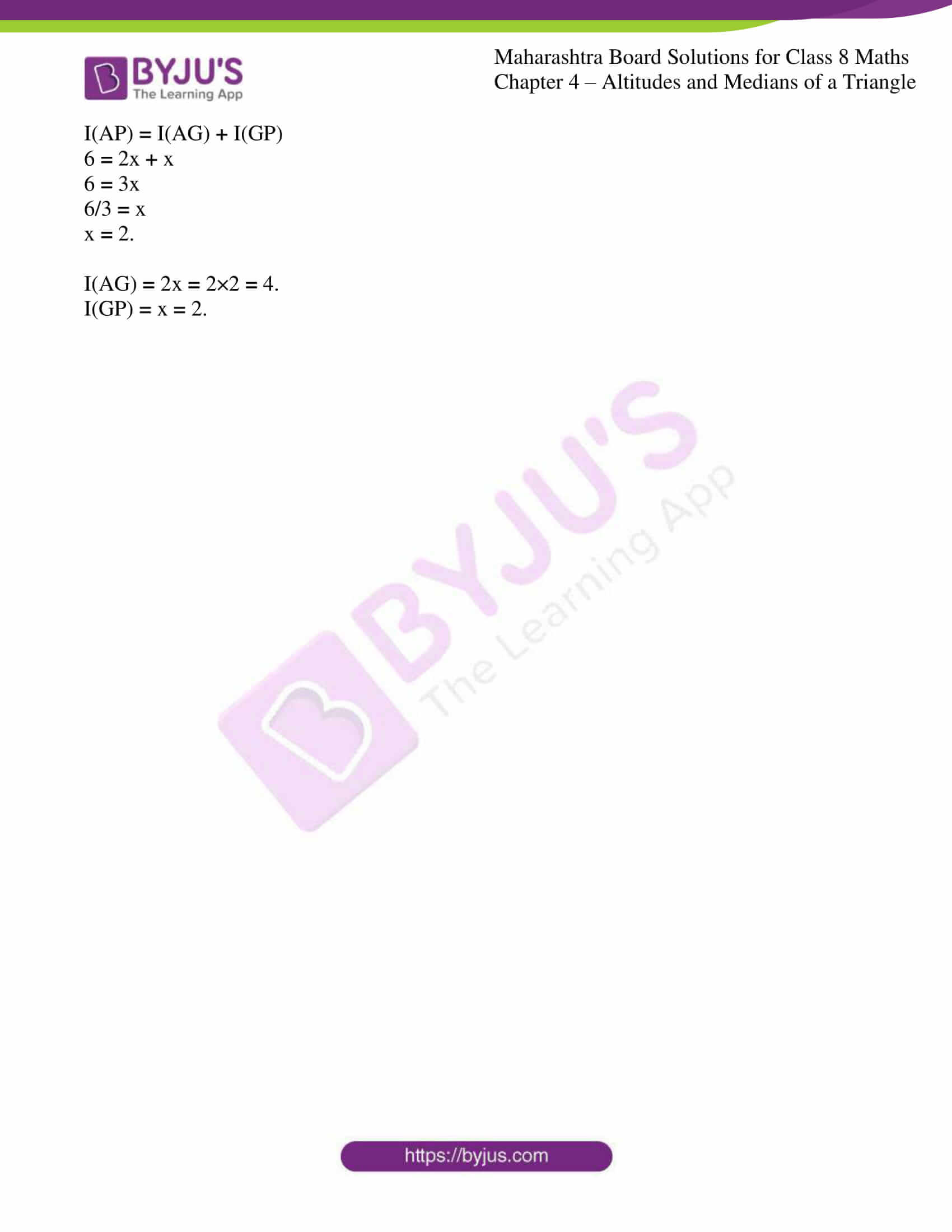### Access answers to Maharashtra Board Solutions For Class 8 Maths Part 1 Chapter 4- Altitudes and Medians of a Triangle.

Practice set 4.1 PAGE NO: 22

1. In ∆LMN, …… is an altitude and …… is a median. (Write the names of appropriate segments.)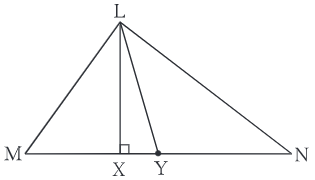Solution:

In ∆LMN, LX is the altitude (since it makes a 90° angle) and LY is a median (since it divides the base into two equal halves i.e., MY = NY).

2. Draw an acute-angled ∆PQR. Draw all of its altitudes. Name the point of concurrence as ‘O’.

Solution:

Here, is the acute-angled ∆PQR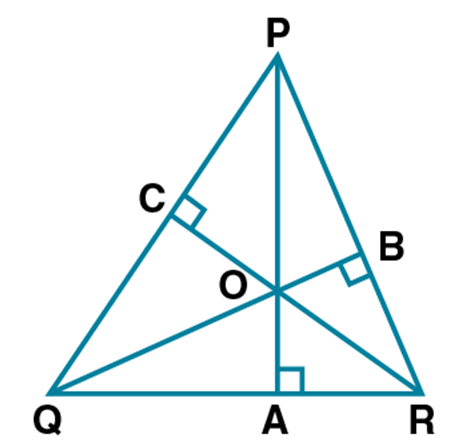Seg PA, seg BQ, seg CR are the altitudes of ∆PQR. The point of concurrence is denoted by the point O.

3. Draw an obtuse-angled ∆STV. Draw its medians and show the centroid.

Solution:

Here, is the obtuse-angled ∆STV.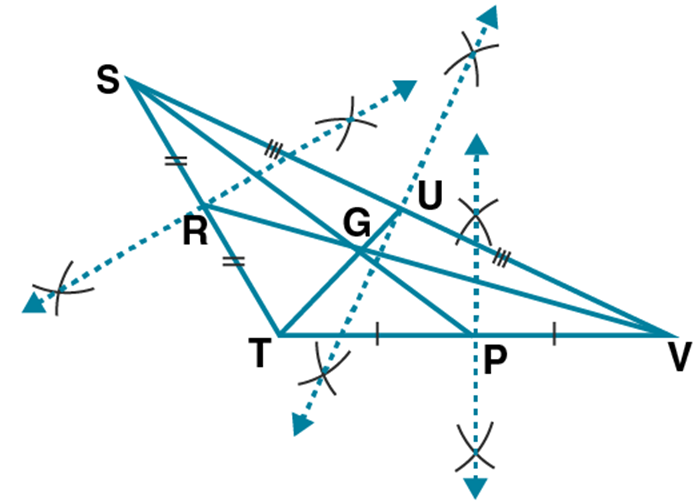Seg SP, seg UT and seg RV are medians of ∆STV.

Their point of concurrence is denoted by G.

4. Draw an obtuse-angled ∆LMN. Draw its altitudes and denote the orthocenter by ‘O’.

Solution:

Here, is the obtuse-angled LMN.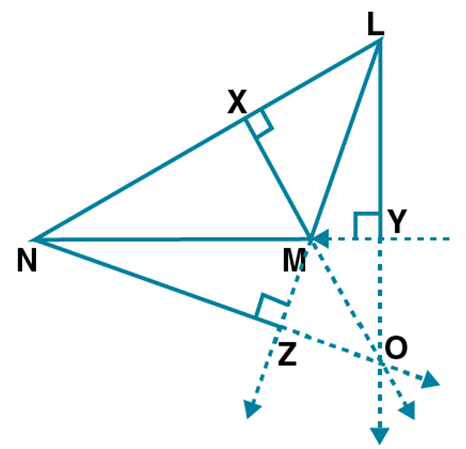The orthocenter of the obtuse triangle lies outside the triangle.

The point O denotes the orthocenter of the obtuse-angled ∆LMN.

5. Draw a right angled ∆XYZ. Draw its medians and show their point of concurrence by G.

Solution:

Here, is the right angled ∆XYZ.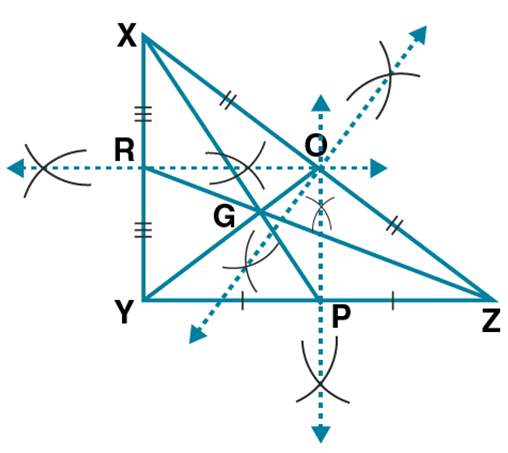Their point of concurrence is denoted by G.

6. Draw an isosceles triangle. Draw all of its medians and altitudes. Write your observation about their points of concurrence.

Solution:

Here, is the isosceles triangle.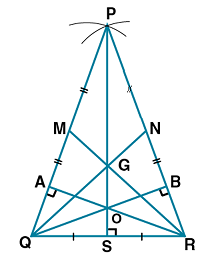The medians i.e. G and altitudes i.e. O lie on the same line on PS which is the perpendicular bisector of seg QR.

7. Fill in the blanks.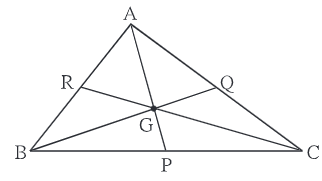Point G is the centroid of ∆ABC.

(1) If l(RG) = 2.5 then l(GC) = ……
(2) If l(BG) = 6 then l(BQ) = ……
(3) If l(AP) = 6 then l(AG) = ….. and l(GP) = …..

Solution:

(1) If l(RG) = 2.5 then l(GC) = 5.

We know that the centroid divides each median in the ratio 2:1.

So, CG/RG = 2/1

CG/2.5 = 2/1

CG = 2 × 2.5

= 5

(2) If l(BG) = 6 then l(BQ) = 9.

We know that the centroid divides each median in the ratio 2:1.

So, BG/QG = 2/1

6/QG = 2/1

6 × 1 = 2 × QG

= 2 × QG

6/2 = QG

QG = 3.

Since we have to find I(BQ), and from the figure it can be seen that,

(BQ) = I(BG) + I(QG)

So, I(BQ) = 6 + 3

I(BQ) = 9.

(3) If l(AP) = 6 then l(AG) = 4 and l(GP) = 2.

We know that the centroid divides each median in the ratio 2:1.

Here both I(AG) and I(GP) are unknown so,

Let I(AG), I(GP) be 2x and x respectively, from equation (i)

I(AP) = I(AG) + I(GP)

= 2x + x

= 3x

6/3 = x

= 2.

I(AG) = 2x = 2×2 = 4.

I(GP) = x = 2.

As the Chapter is about Altitudes and Medians of a Triangle, one must be able to develop an interest and strengthen the foundation of the subject. Regular revision of important concepts and formulas over time is the best way to strengthen your concepts.

Many such exercise problems are given in the MSBSHSE Solutions book. Students can go through those solutions on a daily basis to improve their performance in the examination.

## Frequently Asked Questions on Maharashtra State Board Solutions for Class 8 Maths Chapter 4 Altitudes and Medians of a Triangle

### Are these Maharashtra State Board Class 8 Maths Chapter 4 Solutions useful to prepare for the exams?

Yes, these Maharashtra State Board Class 8 Maths Chapter 4 Solutions are very helpful. Students are recommended to practise these solutions after revising the subjects because they set the basis for the questions that could get asked often in the board exams.